15 49.0138 8.38624 1 1 4000 1 https://valorant-update.com 300 true 0# The BEST TEAMMATE In Pro Valorant

So far we have the settings for:

• 100T Asuna
• Brax
• Faze Babybay
• 100T Hiko
• G2 Mixwell
• 100T Nitro
• Noted
• TL Scream
• Shanks
• Shroud
• SEN Sinatraa
• TSM Subroza
• Tenz
• TSM Wardell

We’ll be continually updating this overtime so be sure to check back often. Let us know in the comments who you’d like to see next!

### 100T Asuna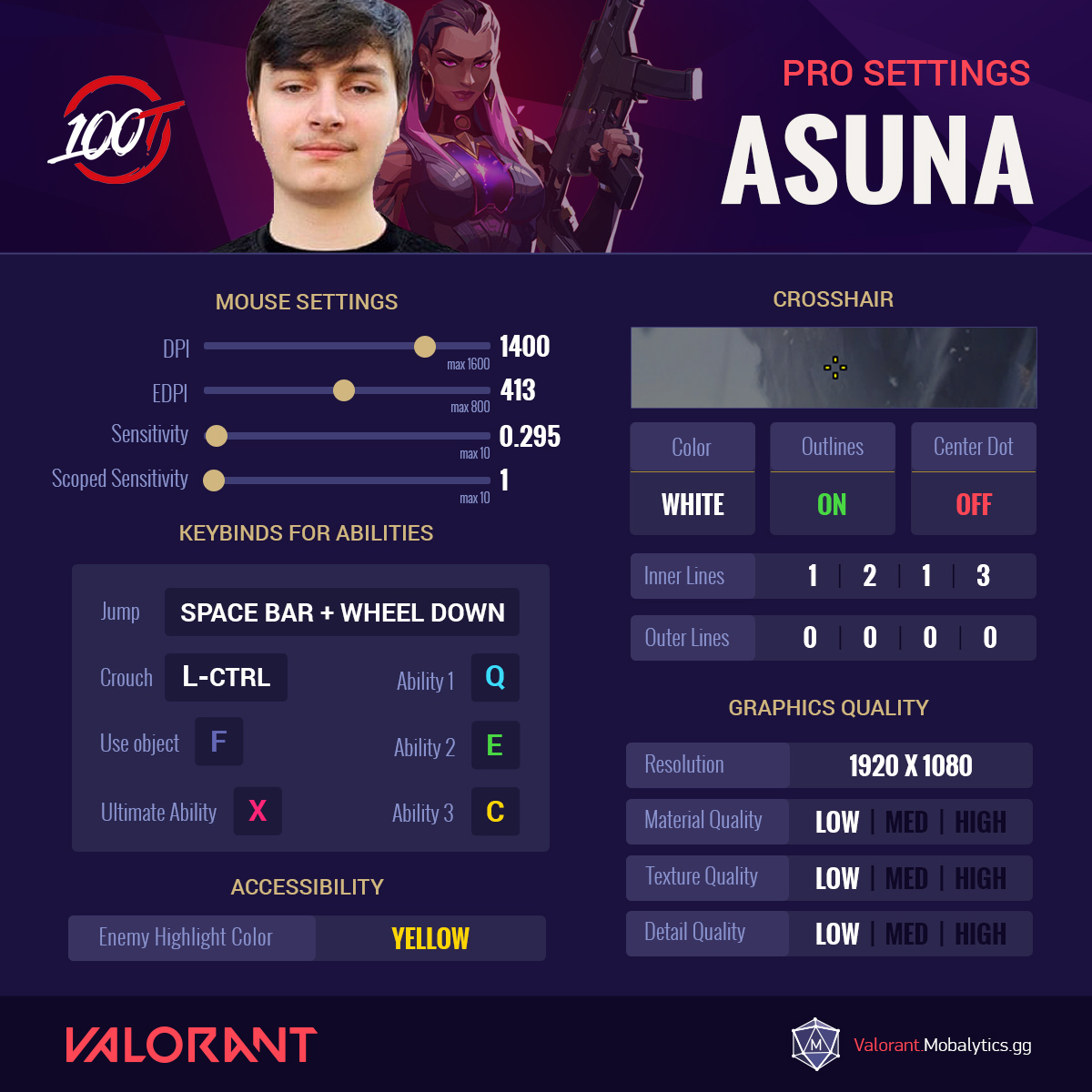#### 100T Asuna’s Valorant Settings

• Mouse Settings
• DPI = 1400 (max 1600)
• EDPI = 413 (max 800)
• Sensitivity =0.295 (max 10)
• Scoped Sensitivity = 1 (max 10)
• Ability Keybinds
• Jump = Spacebar + Wheel Down
• Crouch = Left Ctrl
• Use Object = F
• Ability 1 = Q
• Ability 2 = E
• Ability 3 = C
• Ultimate Ability = X
• Crosshair
• Color = White
• Outlines = On
• Center Dot = Off
• Inner Lines = 1|2|1|3
• Outer Lines = 0|0|0|0
• Graphics Quality
• Resolution = 1920 x 1080
• Material Quality = Low
• Texture Quality = Low
• Detail Quality = Low
• Accessibility
• Enemy Highlight Color = Yellow.

### Babybay (Faze)#### Babybay’s Valorant Settings

• Mouse Settings
• DPI = 800 (max 1600)
• EDPI = 195.2 (max 800)
• Sensitivity =0.244 (max 10)
• Scoped Sensitivity = 1 (max 10)
• Ability Keybinds
• Jump = Spacebar
• Crouch = Left Ctrl
• Use Object = F
• Ability 1 = E
• Ability 2 = Q
• Ability 3 = C
• Ultimate Ability = X
• Crosshair
• Color = White
• Outlines = Off
• Center Dot = Off
• Inner Lines = 1|4|2|2
• Outer Lines = 0|0|0|0
• Graphics Quality
• Resolution = 1920 x 1080
• Material Quality = Med
• Texture Quality = Med
• Detail Quality = Med
• Accessibility
• Enemy Highlight Color = Yellow.

### Brax (fomerly T1)#### Brax’s Valorant Settings

• Mouse Settings
• DPI = 400 (max 1600)
• EDPI = 136 (max 800)
• Sensitivity =0.34 (max 10)
• Scoped Sensitivity = 1 (max 10)
• Ability Keybinds
• Jump = Spacebar
• Crouch = Left Ctrl
• Use Object = F
• Ability 1 = E
• Ability 2 = Q
• Ability 3 = C
• Ultimate Ability = X
• Crosshair
• Color = Green
• Outlines = On
• Center Dot = Off
• Inner Lines = 1|4|3|4
• Outer Lines = 1|0|2|0
• Graphics Quality
• Resolution = 1600 x 900
• Note that Brax has recently changed to full HD
• Material Quality = Low
• Texture Quality = Low
• Detail Quality = Low
• Accessibility
• Enemy Highlight Color = Red

### Hiko (100 Thieves)#### 100T Hiko’s Valorant Settings

• Mouse Settings
• DPI = 1600 (max 1600)
• EDPI = 576 (max 800)
• Sensitivity = 0.36 (max 10)
• Scoped Sensitivity = 1 (max 10)
• Ability Keybinds
• Jump = Spacebar
• Crouch = Left Ctrl
• Use Object = F
• Ability 1 = C
• Ability 2 = Q
• Ability 3 = E
• Ultimate Ability = X
• Crosshair
• Color = Green
• Outlines = On
• Center Dot = On
• Inner Lines = 1|4|2|3
• Outer Lines = 0|0|6|8
• Graphics Quality
• Resolution = 1920 x 1080
• Material Quality = High
• Texture Quality = High
• Detail Quality = High
• Accessibility
• Enemy Highlight Color = Red

####### G2 Mixwell’s Valorant Settings

• Mouse Settings
• DPI = 400 (max 1600)
• EDPI = 276 (max 800)
• Sensitivity = 0.69 (max 10)
• Scoped Sensitivity = 1 (max 10)
• Ability Keybinds
• Jump = Spacebar + Wheel Down
• Crouch = Left Ctrl
• Use Object = F
• Ability 1 = Q
• Ability 2 = E
• Ability 3 = C
• Ultimate Ability = X
• Crosshair
• Color = White
• Outlines = Off
• Center Dot = Off
• Inner Lines = 1|4|2|3
• Outer Lines = 0|0|0|0
• Graphics Quality
• Resolution = 1920 x 1080
• Material Quality = Low
• Texture Quality = Low
• Detail Quality = Low
• Accessibility
• Enemy Highlight Color = Red.

### Nitro (100 Thieves)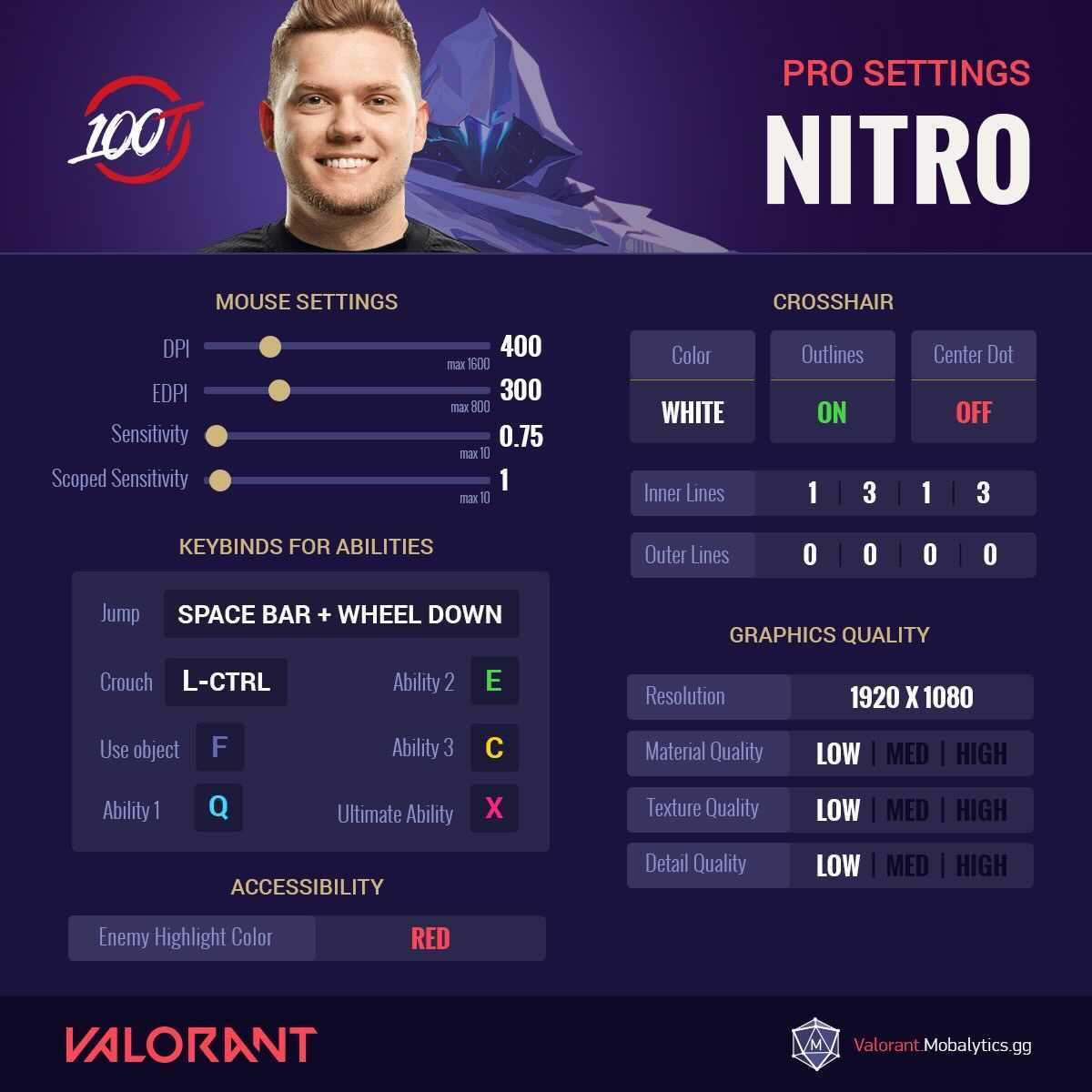#### 100T Nitro’s Valorant Settings

• Mouse Settings
• DPI = 400 (max 1600)
• EDPI = 300 (max 800)
• Sensitivity = 0.75 (max 10)
• Scoped Sensitivity = 1 (max 10)
• Ability Keybinds
• Jump = Spacebar + Wheel Down
• Crouch = Left Ctrl
• Use Object = F
• Ability 1 = Q
• Ability 2 = E
• Ability 3 = C
• Ultimate Ability = X
• Crosshair
• Color = White
• Outlines = Off
• Center Dot = On
• Inner Lines = 1|3|1|3
• Outer Lines = 0|0|0|0
• Graphics Quality
• Resolution = 1920 x 1080
• Material Quality = Low
• Texture Quality = Low
• Detail Quality = Low
• Accessibility
• Enemy Highlight Color = Red.

### Noted (Streamer)#### Noted’s Valorant Settings

• Mouse Settings
• DPI = 800 (max 1600)
• EDPI = 388.8 (max 800)
• Sensitivity = 0.486(max 10)
• Scoped Sensitivity = 1 (max 10)
• Ability Keybinds
• Jump = Spacebar + Wheel Down
• Crouch = Left Ctrl
• Use Object = F
• Ability 1 = Q
• Ability 2 = E
• Ability 3 = C
• Ultimate Ability = X
• Crosshair
• Color = Yellow
• Outlines = Off
• Center Dot = Off
• Inner Lines = 1|3|2|1
• Outer Lines = 0|0|0|0
• Graphics Quality
• Resolution = 1920 x 1080
• Material Quality = Low
• Texture Quality = Low
• Detail Quality = Low
• Accessibility
• Enemy Highlight Color = Yellow.

### Sinatraa (SEN)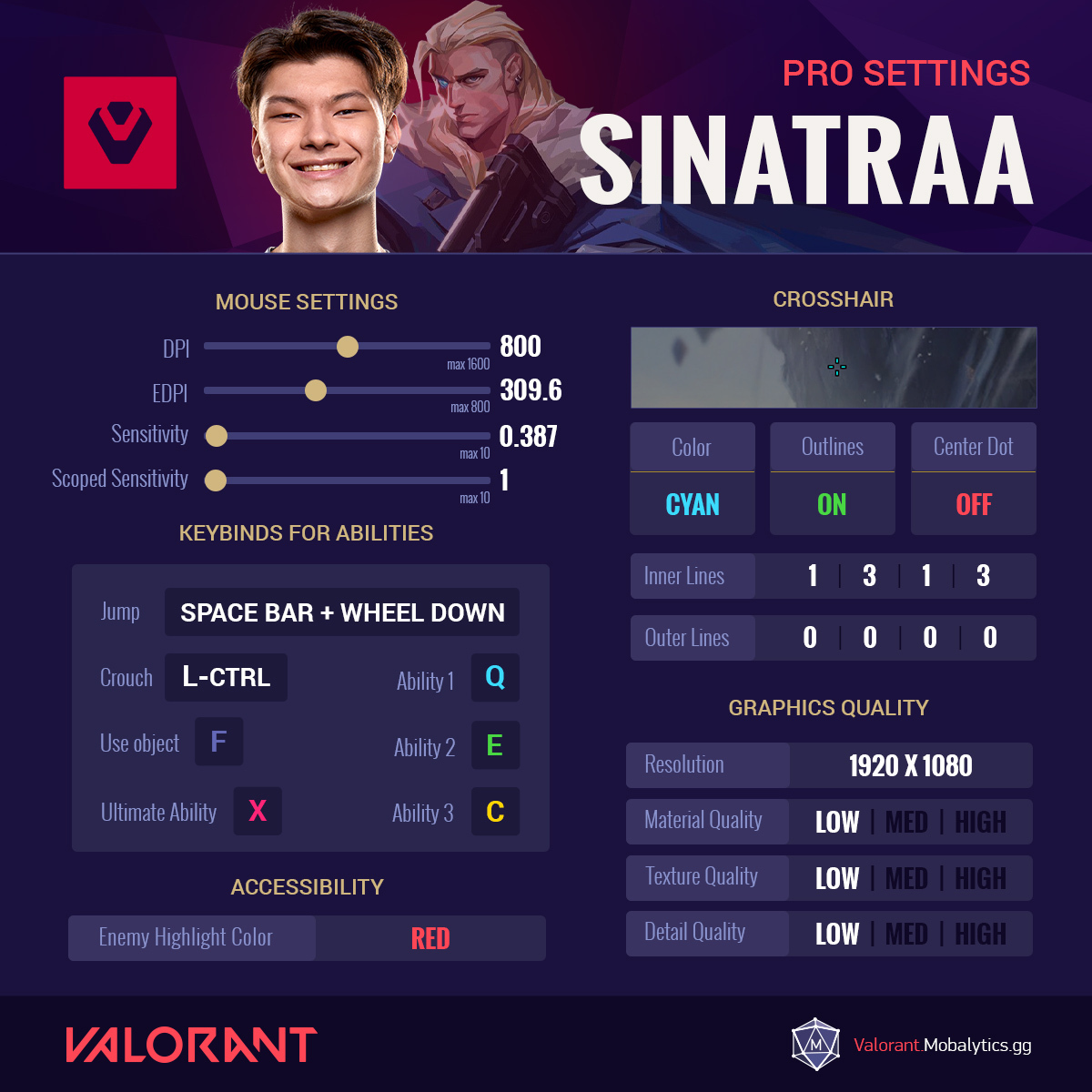#### SEN Sinatraa’s Valorant Settings

• Mouse Settings
• DPI = 800 (max 1600)
• EDPI = 309.6 (max 800)
• Sensitivity = 0.387(max 10)
• Scoped Sensitivity = 1 (max 10)
• Ability Kybinds
• Jump = Spacebar + Wheel Down
• Crouch = Left Ctrl
• Use Object = F
• Ability 1 = Q
• Ability 2 = E
• Ability 3 = C
• Ultimate Ability = X
• Crosshair
• Color = Cyan
• Outlines = On
• Center Dot = Off
• Inner Lines = 1|3|1|3
• Outer Lines = 0|0|0|0
• Graphics Quality
• Resolution = 1920 x 1080
• Material Quality = Low
• Texture Quality = Low
• Detail Quality = Low
• Accessibility
• Enemy Highlight Color = Red.

### Subroza (TSM)#### TSM Subroza’s Valorant Settings

• Mouse Settings
• DPI = 800 (max 1600)
• EDPI = 221.6 (max 800)
• Sensitivity = 0.277(max 10)
• Scoped Sensitivity = 1 (max 10)
• Ability Keybinds
• Jump = Spacebar + Wheel Down
• Crouch = Left Ctrl
• Use Object = E
• Ability 1 = MB4
• Ability 2 = MB3
• Ability 3 = MB5
• Ultimate Ability = C
• Crosshair
• Color = Red
• Outlines = On
• Center Dot = Off
• Inner Lines = 1|1|4|2
• Outer Lines = 1|3|1|3
• Graphics Quality
• Resolution = 1920 x 1080
• Material Quality = Low
• Texture Quality = Low
• Detail Quality = Low
• Accessibility
• Enemy Highlight Color = Yellow

### Scream (Team Liquid)#### TL Screams’s Valorant Settings

• Mouse Settings
• DPI = 400 (max 1600)
• EDPI = 306 (max 800)
• Sensitivity =0.765 (max 10)
• Scoped Sensitivity = 1 (max 10)
• Ability Keybinds
• Jump = Spacebar
• Crouch = Left Ctrl
• Use Object = E
• Ability 1 = F
• Ability 2 = Q
• Ability 3 = C
• Ultimate Ability = X
• Crosshair
• Color = Cyan
• Outlines = On
• Center Dot = On
• Inner Lines = 1|0|0|0
• Outer Lines = 0|0|0|0
• Graphics Quality
• Resolution = 1920 x 1080
• Material Quality = Low
• Texture Quality = Low
• Detail Quality = Low
• Accessibility
• Enemy Highlight Color = Red.

### Shanks (Streamer)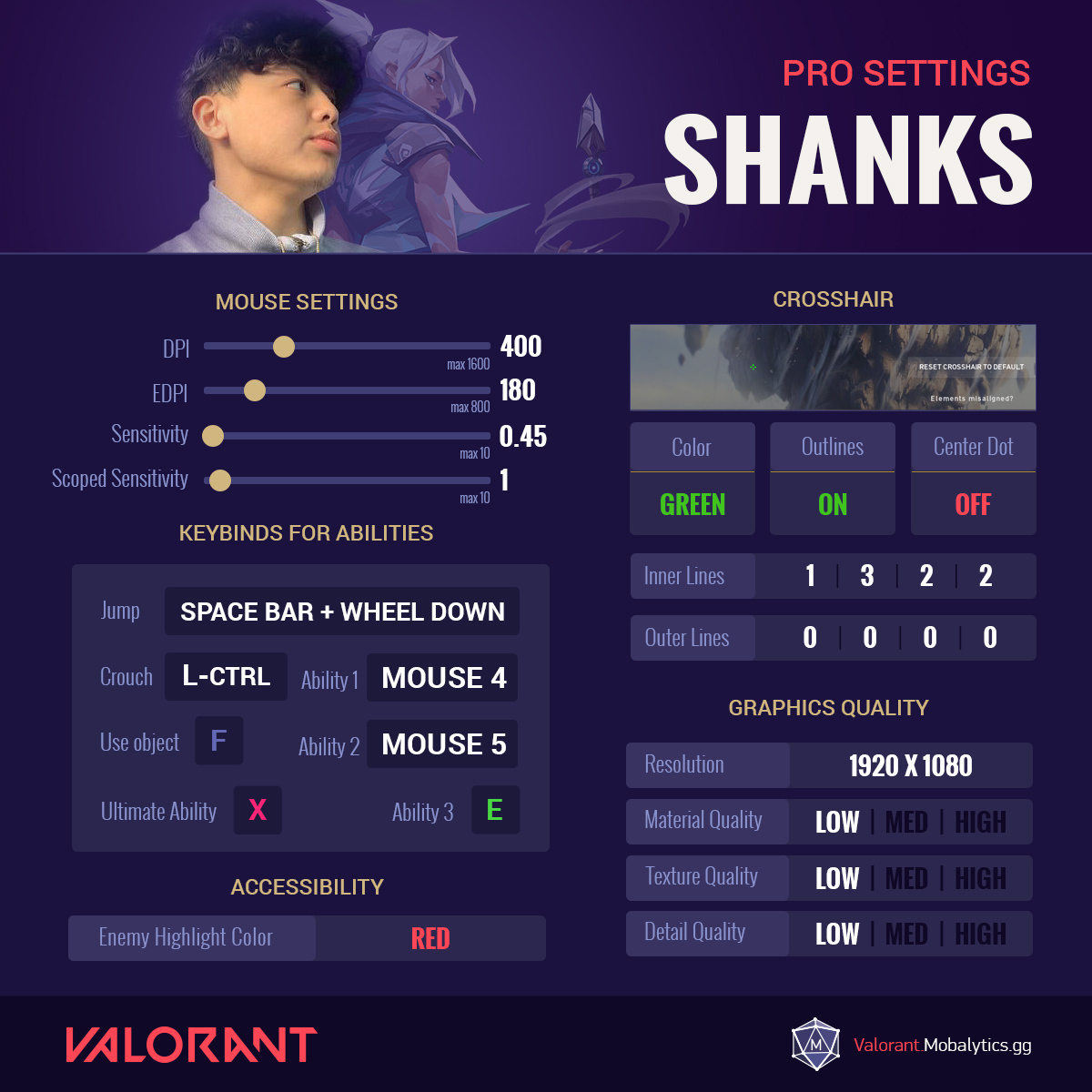#### Shanks’s Valorant Settings

• Mouse Settings
• DPI = 400 (max 1600)
• EDPI = 180 (max 800)
• Sensitivity =0.45 (max 10)
• Scoped Sensitivity = 1 (max 10)
• Ability Keybinds
• Jump = Spacebar + wheel down
• Crouch = Left Ctrl
• Use Object = F
• Ability 1 = Mouse 4
• Ability 2 = Mouse 5
• Ability 3 = E
• Ultimate Ability = X
• Crosshair
• Color = Green
• Outlines = On
• Center Dot = Off
• Inner Lines = 1|3|2|2
• Outer Lines = 0|0|0|0
• Graphics Quality
• Resolution = 1920 x 1080
• Material Quality = Low
• Texture Quality = Low
• Detail Quality = Low
• Accessibility
• Enemy Highlight Color = Red

### Shroud (Streamer)#### Shroud’s Valorant Settings

• Mouse Settings
• DPI = 450 (max 1600)
• EDPI = 351 (max 800)
• Sensitivity =0.78 (max 10)
• Scoped Sensitivity = 1 (max 10)
• Ability Keybinds
• Jump = Spacebar
• Crouch = Left Ctrl
• Use Object = F
• Ability 1 = E
• Ability 2 = Q
• Ability 3 = C
• Ultimate Ability = X
• Crosshair
• Color = Cyan
• Outlines = Off
• Center Dot = On
• Inner Lines = 1|5|4|5
• Outer Lines = 1|5|4|5
• Graphics Quality
• Resolution = 1920 x 1080
• Material Quality = High
• Texture Quality = High
• Detail Quality = High
• Accessibility
• Enemy Highlight Color = Red

### Tenz (SEN)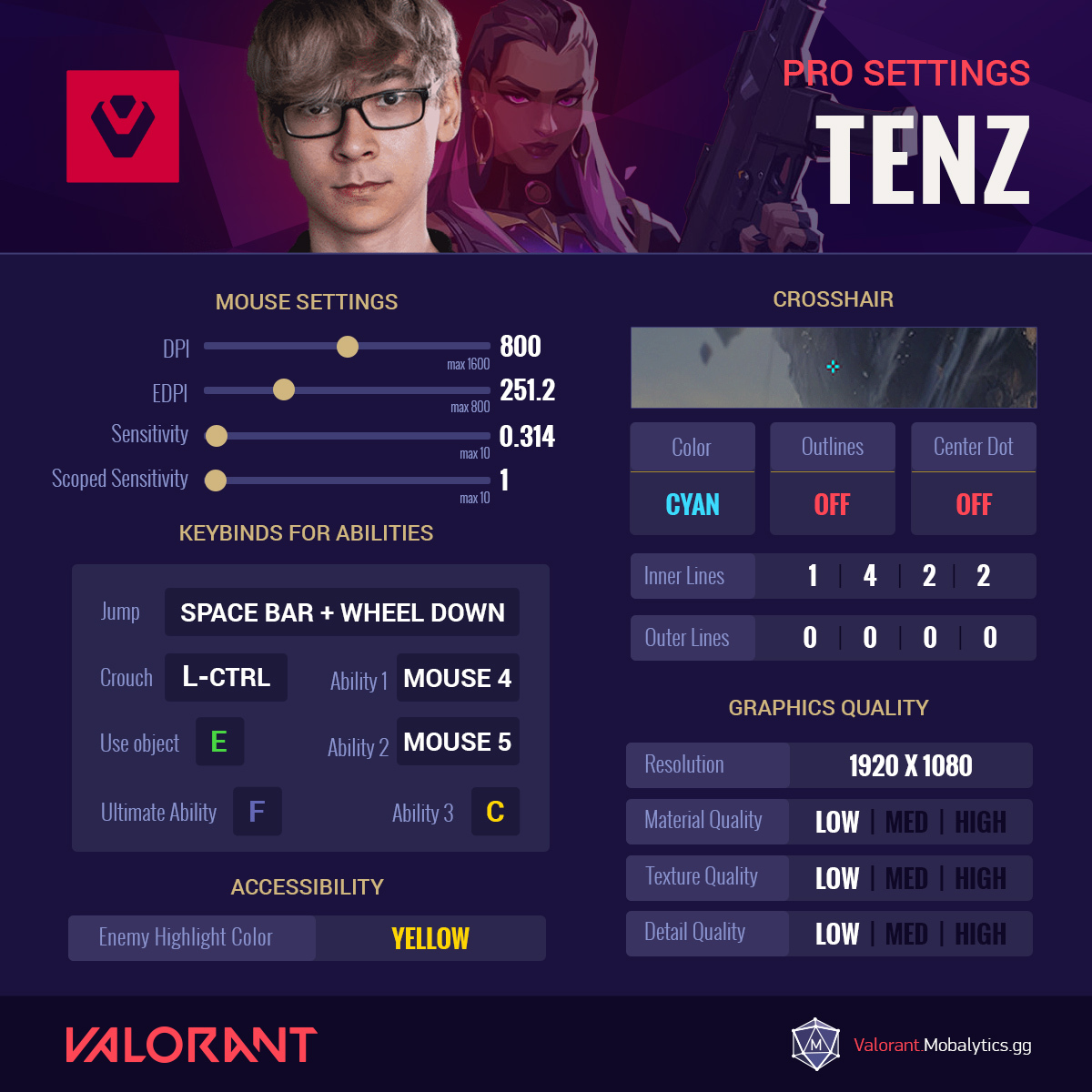#### SEN Tenz’s Valorant Settings

• Mouse Settings
• DPI = 800 (max 1600)
• EDPI = 251.2 (max 800)
• Sensitivity = 0.314 (max 10)
• Scoped Sensitivity = 1 (max 10)
• Ability Keybinds
• Jump = Spaec Bar + Wheel Down
• Crouch = Left Ctrl
• Use Object = E
• Ability 1 = Mouse 4
• Ability 2 = Mouse 5
• Ability 3 = C
• Ultimate Ability = F
• Crosshair
• Color = Cyan
• Outlines = Off
• Center Dot = Off
• Inner Lines = 1|4|2|2
• Outer Lines = 0|0|0|0
• Graphics Quality
• Resolution = 1920 x 1080
• Material Quality = Low
• Texture Quality = Low
• Detail Quality = Low
• Accessibility
• Enemy Highlight Color = Yellow.

### Scream (Team Liquid)#### TSM Wardell’s Valorant Settings

• Mouse Settings
• DPI = 400 (max 1600)
• EDPI = 261.2 (max 800)
• Sensitivity =0.653(max 10)
• Scoped Sensitivity = 1.15 (max 10)
• Ability Keybinds
• Jump = Wheel Down
• Crouch = Left Ctrl
• Use Object = E
• Ability 1 = Mouse 4
• Ability 2 = Mouse 5
• Ability 3 = F
• Ultimate Ability = C
• Crosshair
• Color = Cyan
• Outlines = Off
• Center Dot = Off
• Inner Lines = 1|4|2|2
• Outer Lines = 0|0|0|0
• Graphics Quality
• Resolution = 1920 x 1080
• Material Quality = Low
• Texture Quality = Low
• Detail Quality = Low
• Accessibility
• Enemy Highlight Color = Yellow.